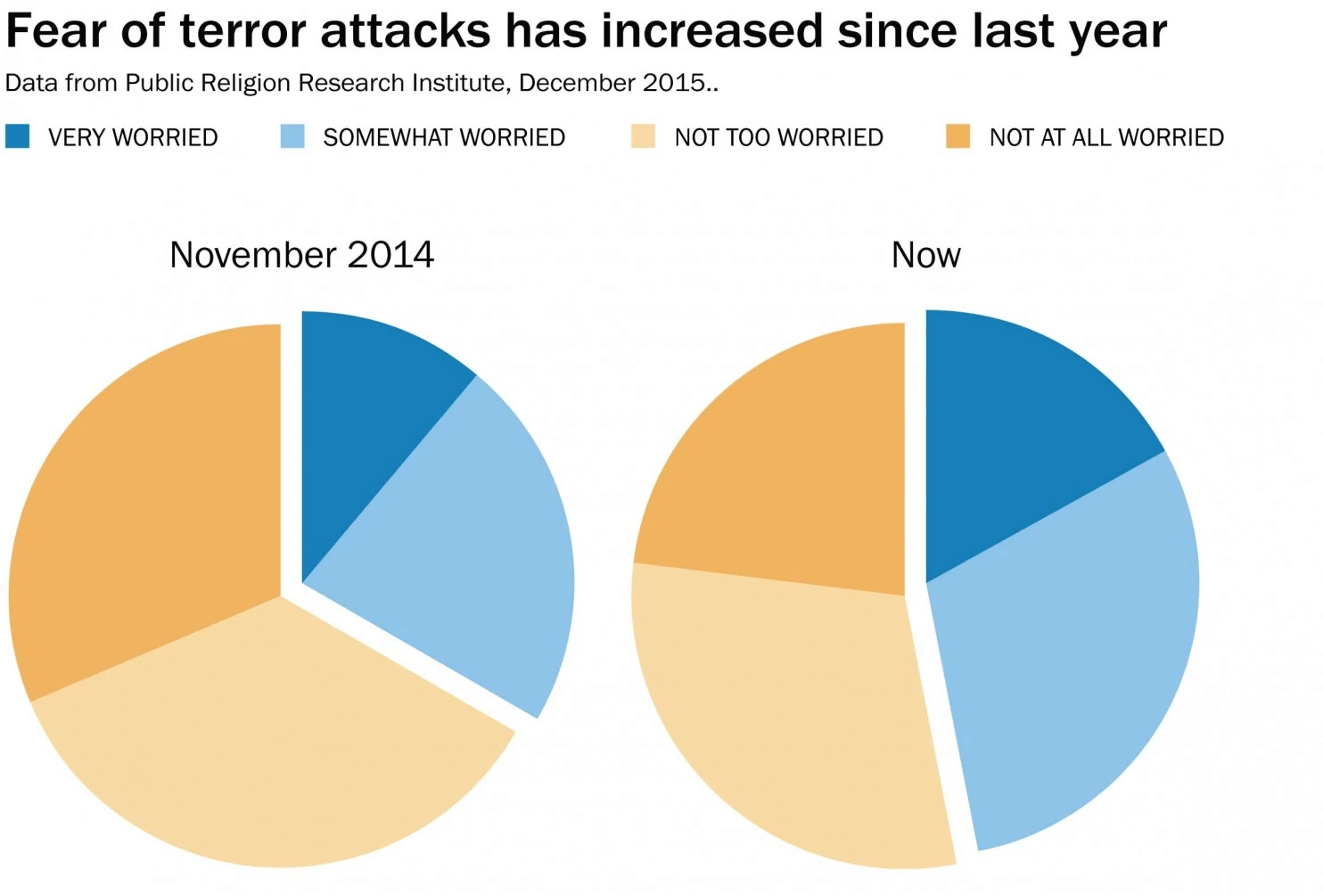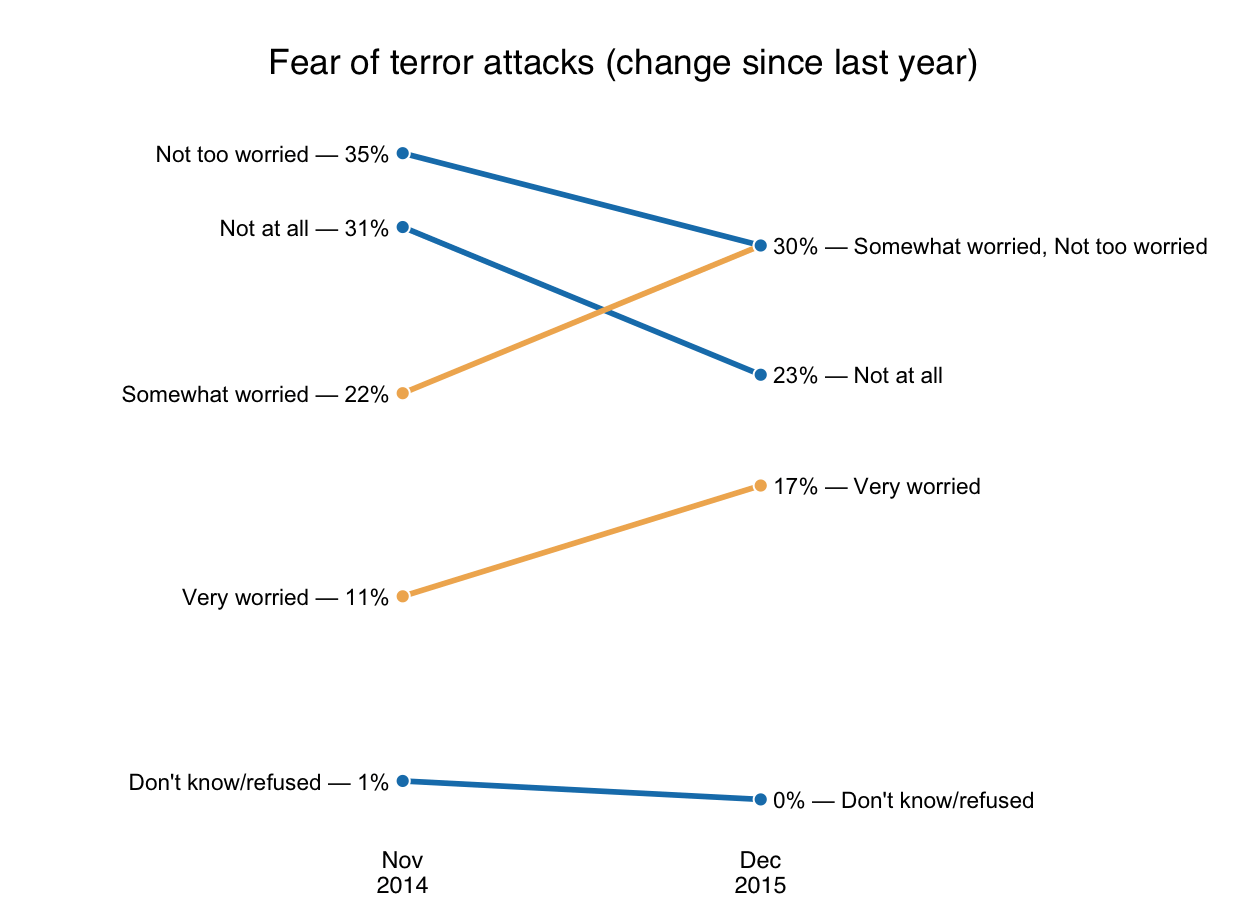Want to share your content on R-bloggers? click here if you have a blog, or here if you don't.

I woke up this morning to a headline story from the Washington Post on “Americans are twice as willing to distance Christian extremists from their religion as Muslims“. This post is not about the content of the headline or story. It is about the horrible pie chart WaPo led the article with:This isn’t just a rant of a madman against pie charts. While I am vehemently opposed to them, we did cover them in our book and my co-author (@jayjacobs) and the incredibly talented @annkemery both agree there are often cases where they are appropriate. Even using their less-sensitive sensibilities, this would not be one of those cases.

So, what—exactly—is the problem? WaPo tried to enable comparison between pies by exploding them and using colors to indicate similar fear levels, mapping shades to entries in the top legend. Your eye has to move around a bit to take everything in and remember the mapping as you focus on each slice (since you will end up doing that given that each category colored differently). Their whole goal was to enable the reader to see the change in sentiment towards terrorism since this time last year.

Hrm. Two dates. Small set of values. Desire to quickly compare change in value/slope. This sounds like a job for a slopegraph!

The article and graphic are based on a survey. Thankfully the complete survey data was made available, which made it easy to do a makeover (in R of course). Here’s the result:Each category change is clearly visible, you don’t need to remember color association and you even know the actual values*.

The R code is below and in this gist. How would you make the WaPo chart better (drop a note in the comments with a link to your own makeover)?

``` library(tidyr) library(ggplot2) library(ggthemes) library(scales) library(dplyr)   # Easiest way to transcribe the PDF table # The slope calculation will enable us to color the lines/points based on up/down dat <- data_frame(`2014-11-01`=c(0.11, 0.22, 0.35, 0.31, 0.01), `2015-12-01`=c(0.17, 0.30, 0.30, 0.23, 0.00), slope=factor(sign(`2014-11-01` - `2015-12-01`)), fear_level=c("Very worried", "Somewhat worried", "Not too worried", "Not at all", "Don't know/refused"))   # Transform that into something we can use dat <- gather(dat, month, value, -fear_level, -slope)   # We need real dates for the X-axis manipulation dat <- mutate(dat, month=as.Date(as.character(month)))   # Since 2 categories have the same ending value, we need to # take care of that (this is one of a few "gotchas" in slopegraph preparation) end_lab <- dat %>% filter(month==as.Date("2015-12-01")) %>% group_by(value) %>% summarise(lab=sprintf("%s", paste(fear_level, collapse=", ")))   gg <- ggplot(dat)   # line gg <- gg + geom_line(aes(x=month, y=value, color=slope, group=fear_level), size=1) # points gg <- gg + geom_point(aes(x=month, y=value, fill=slope, group=fear_level), color="white", shape=21, size=2.5)   # left labels gg <- gg + geom_text(data=filter(dat, month==as.Date("2014-11-01")), aes(x=month, y=value, label=sprintf("%s — %s ", fear_level, percent(value))), hjust=1, size=3) # right labels gg <- gg + geom_text(data=end_lab, aes(x=as.Date("2015-12-01"), y=value, label=sprintf(" %s — %s", percent(value), lab)), hjust=0, size=3)   # Here we do some slightly tricky x-axis formatting to ensure we have enough # space for the in-panel labels, only show the months we need and have # the month labels display properly gg <- gg + scale_x_date(expand=c(0.125, 0), labels=date_format("%bn%Y"), breaks=c(as.Date("2014-11-01"), as.Date("2015-12-01")), limits=c(as.Date("2014-02-01"), as.Date("2016-12-01"))) gg <- gg + scale_y_continuous()   # I used colors from the article gg <- gg + scale_color_manual(values=c("#f0b35f", "#177fb9")) gg <- gg + scale_fill_manual(values=c("#f0b35f", "#177fb9")) gg <- gg + labs(x=NULL, y=NULL, title="Fear of terror attacks (change since last year)n") gg <- gg + theme_tufte(base_family="Helvetica") gg <- gg + theme(axis.ticks=element_blank()) gg <- gg + theme(axis.text.y=element_blank()) gg <- gg + theme(legend.position="none") gg <- gg + theme(plot.title=element_text(hjust=0.5)) gg ```

* Well, it’s survey. To add insult to injury, it’s a sentiment-based survey given right after a likely-to-be-attributed-terrorism attack. Also, there is a margin of error that isn’t communicated in either visualization. So while there is “data”, trust it at your own peril.